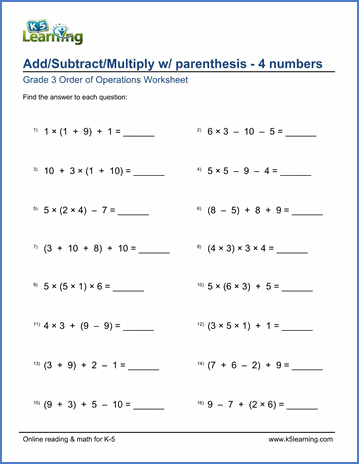# Grade 3 Order of Operations Worksheets

## Order of operations worksheets

These worksheets provide practice in the basic rules for the order in which arithmetic operations are performed.  Operations within parenthesis are performed prior to operations outside of parenthesis, and multiplications are done prior to additions or subtractions.  There is no use of exponents or negative numbers in these questions.

## Order of Operations

Example

23 - (3 + 13) =
23 - (3 + 13 - 6) =
(33+9) + 4 - (30+15) =
(33+9) + 4 - (35-3+1) =

7 + 4 x 4 - 10 =
4 + 3 x (4 + 10) =
3+21 x 6 + (24-4) =

3+21 x 6 + (24-4) x 2 =Sample Grade 3 Order of Operations Worksheet

## More order of operations worksheets

Browse all of our order of operations worksheets, from adding and subtracting with parenthesis to full PEMDAS worksheets including exponents.

## What is K5?

K5 Learning offers reading and math worksheets, workbooks and an online reading and math program for kids in kindergarten to grade 5.  We help your children build good study habits and excel in school.